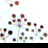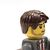# arcrest geometryfilter?

3399
2
05-30-2016 07:09 PMNew Contributor II

Is there a better way of getting that geometry into the second query? Not happy with line 28

```from __future__ import print_function
from arcrest.ags import FeatureLayer
import arcpy
import json

if __name__ == "__main__":

arcpy.env.overwriteOutput = True

flLGA = FeatureLayer(
url=lgaUrl)

lgaWhereClause = "NAME = 'Launceston'"
outSR = '28355'
##    outFC="C:\Scripts\data.gdb\LGA"
##    # get lga geometry
##    lgaGeom = flLGA.query(where=lgaWhereClause,out_fields='shape',returnGeometry=True,returnFeatureClass=True,out_fc=outFC,outSR=outSR)
lgaGeom = flLGA.query(where=lgaWhereClause,out_fields='shape',returnGeometry=True,outSR=outSR)
geomText = lgaGeom.toJSON

for key, value in geomRings.iteritems():
if key == 'features':
features = str(value)

features = features.replace("[{u'geometry': ","").replace(", u'attributes': {}}]","").replace("{u'rings':","{'rings':")

flCrown = FeatureLayer(
url=crownUrl)

crownWhereClause = "1=1"
geometryType = "esriGeometryPolygon"

38.

flCrown.query(where=crownWhereClause,geometry=features,out_fields='objectid,pid,shape',returnGeometry=Tr

e,returnFeatureClass=True,out_fc=outFC,geometryType=geometryType,inSR=outSR,outSR=outSR)

1 Solution

Accepted SolutionsbyEsri Regular Contributor

Try this:

``````import arcrest
if __name__ == "__main__":
org_url="https://site.com/arcgis"
where = "ISO_CC = 'US'"
org_url=org_url,
token_url=token_url)
fl = arcrest.ags.FeatureLayer(url=fl_url,securityHandler=sh)
fs = fl.query(where=where)
print ("Found %s features with the where clause: %s" % (len(fs), where) )
if len(fs.features) > 0:
feature = fs.features
geom = feature.geometry
geomFilter = arcrest.filters.GeometryFilter(geomObject=geom)
result = fl.query(where=where, geomtryFilter=geomFilter, as_json=True) # set as_json=False for the featureset object
print ("Found %s features with the where clause: %s and geometry filter" % (len(result['features']), where) )``````
2 RepliesbyMVP Esteemed Contributor

Have you inquired about this at the ArcREST GitHub site?  If so, what was the response?  If not, it might be worth asking there as well while you wait for feedback here.byEsri Regular Contributor

Try this:

``````import arcrest
if __name__ == "__main__":
org_url="https://site.com/arcgis"
where = "ISO_CC = 'US'"